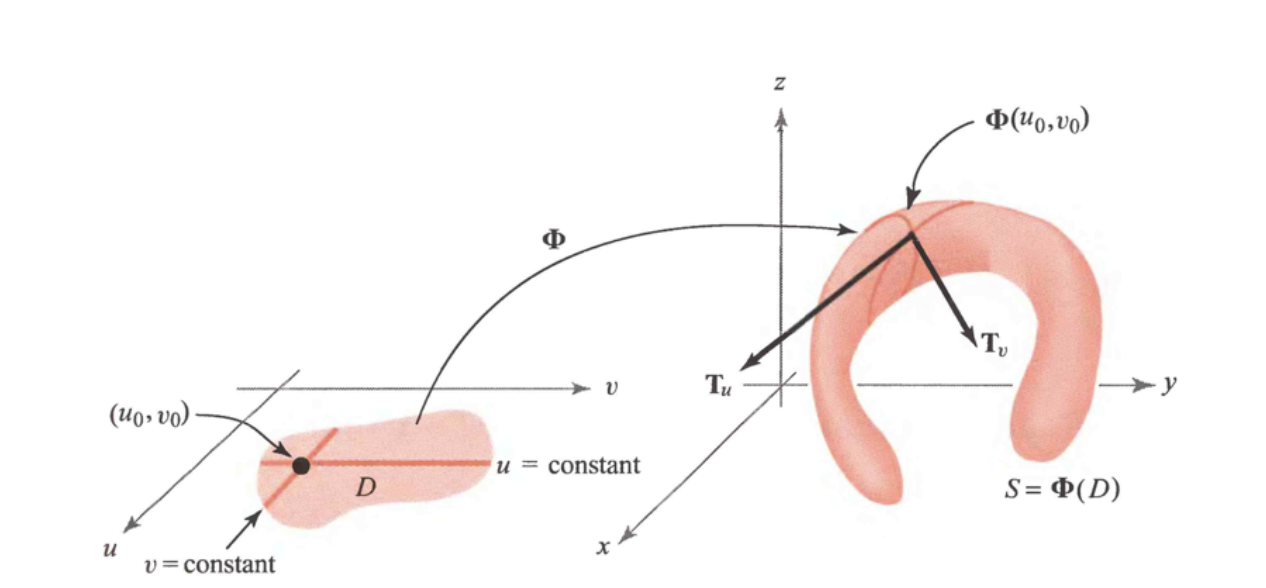# Methodology

## 1. Tangent Vectors to Parametrized Surfaces

Suppose that $\Phi$ is a parametrized surface that is differentiable at $\left(u_{0}, v_{0}\right) \in \mathbb{R}^{2}$.Figure 1. The tangent vectors $\mathbf{T}_{u}$ and $\mathbf{T}_{v}$ that are tangent to the curve on a surface $S,$ and hence tangent to $S$

Fixing $u$ at $u_{0},$ we get a $\operatorname{map} \mathbb{R} \rightarrow \mathbb{R}^{3}$ given by $t \mapsto \boldsymbol{\Phi}\left(u_{0}, t\right),$ whose image is a curve on the surface.

the vector tangent to this curve at the point $\Phi\left(u_{0}, v_{0}\right),$ which we denote by $\mathbf{T}_{v},$ is given by

$\mathbf{T}_{v}=\frac{\partial \boldsymbol{\Phi}}{\partial v}=\frac{\partial x}{\partial v}\left(u_{0}, v_{0}\right) \mathbf{i}+\frac{\partial y}{\partial v}\left(u_{0}, v_{0}\right) \mathbf{j}+\frac{\partial z}{\partial v}\left(u_{0}, v_{0}\right) \mathbf{k}$

Similarly, if we fix $v$ and consider the curve $t \mapsto \Phi\left(t, v_{0}\right),$ we obtain the tangent vector to this curve at $\Phi\left(u_{0}, v_{0}\right),$ given by

$\mathbf{T}_{u}=\frac{\partial \boldsymbol{\Phi}}{\partial u}=\frac{\partial x}{\partial u}\left(u_{0}, v_{0}\right) \mathbf{i}+\frac{\partial y}{\partial u}\left(u_{0}, v_{0}\right) \mathbf{j}+\frac{\partial z}{\partial u}\left(u_{0}, v_{0}\right) \mathbf{k}$

We can use the fact that $\mathbf{n}=\mathbf{T}_{u} \times \mathbf{T}_{v}$ is normal to the surface to both formally define the tangent plane and to compute it.

DEFINITION: The Tangent Plane to a Surface

If a parametrized surface $\boldsymbol{\Phi}: D \subset \mathbb{R}^{2} \rightarrow \mathbb{R}^{3}$ is regular at $\Phi\left(u_{0}, v_{0}\right),$ that is, if $\mathbf{T}_{u} \times \mathbf{T}_{v} \neq \mathbf{0}$ at $\left(u_{0}, v_{0}\right),$ we define the tangent plane of the surface at $\Phi\left(u_{0}, v_{0}\right)$ to be the plane determined by the vectors $\mathbf{T}_{u}$ and $\mathbf{T}_{v} .$ Thus, $\mathbf{n}=\mathbf{T}_{u} \times \mathbf{T}_{v}$ is a normal vector, and an equation of the tangent plane at $\left(x_{0}, y_{0}, z_{0}\right)$ on the surface is given by

$\left(x-x_{0}, y-y_{0}, z-z_{0}\right) \cdot \mathbf{n}=0$

where $\mathbf{n}$ is evaluated at $\left(u_{0}, v_{0}\right) ;$ that is, the tangent plane is the set of $(x, y, z)$ satisfying $(1) .$ If $\mathbf{n}=\left(n_{1}, n_{2}, n_{3}\right)=n_{1} \mathbf{i}+n_{2} \mathbf{j}+n_{3} \mathbf{k},$ then formula (1) becomes

$n_{1}\left(x-x_{0}\right)+n_{2}\left(y-y_{0}\right)+n_{3}\left(z-z_{0}\right)=0$

Suppose a surface $S$ is the graph of a differentiable function $g: \mathbb{R}^{2} \rightarrow \mathbb{R} .$

We wish to write $S$ in parametric form and show that the surface is smooth at all points $\left(u_{0}, v_{0}, g\left(u_{0}, v_{0}\right)\right) \in \mathbb{R}^{3}$

We write $S$ in parametric form as follows:

$x=u, \quad y=v, \quad z=g(u, v)$

which is the same as $z=g(x, y) .$ Then at the point $\left(u_{0}, v_{0}\right)$

$\mathbf{T}_{u}=\mathbf{i}+\frac{\partial g}{\partial u}\left(u_{0}, v_{0}\right) \mathbf{k} \quad \text { and } \quad \mathbf{T}_{v}=\mathbf{j}+\frac{\partial g}{\partial v}\left(u_{0}, v_{0}\right) \mathbf{k}$

and for $\left(u_{0}, v_{0}\right) \in \mathbb{R}^{2}$

$\mathbf{n}=\mathbf{T}_{u} \times \mathbf{T}_{v}=-\frac{\partial g}{\partial u}\left(u_{0}, v_{0}\right) \mathbf{i}-\frac{\partial g}{\partial v}\left(u_{0}, v_{0}\right) \mathbf{j}+\mathbf{k} \neq \mathbf{0}$

This is nonzero because the coefficient of $\mathbf{k}$ is $1$ ; consequently, the parametrization $(u, v) \mapsto(u, v, g(u, v))$ is regular at all points.

The tangent plane at the point $\left(x_{0}, y_{0}, z_{0}\right)=\left(u_{0}, v_{0}, g\left(u_{0}, v_{0}\right)\right)$ is given, by formula $(1),$ as

$\left(x-x_{0}, y-y_{0}, z-z_{0}\right) \cdot\left(-\frac{\partial g}{\partial u},-\frac{\partial g}{\partial v}, 1\right)=0$

where the partial derivatives are evaluated at $\left(u_{0}, v_{0}\right) .$

 Remembering that $x=u$ and $y=v,$ we can write this as $z-z_{0}=\left(\frac{\partial g}{\partial x}\right)\left(x-x_{0}\right)+\left(\frac{\partial g}{\partial y}\right)\left(y-y_{0}\right)$ where $\partial g / \partial x$ and $\partial g / \partial y$ are evaluated at $\left(x_{0}, y_{0}\right)$

## 2. Reconstruction from slope data

In this docummentation, we study a method for numerical integration of a gradient field over a 2 D-grid. Our aim is to estimate the values of a function $z: \mathbb{R}^{2} \rightarrow \mathbb{R},$ over a set $\Omega \subset \mathbb{R}^{2}(\text { reconstruction domain })$ where an estimate $\mathbf{d}=$ $[p, q]^{\top}: \Omega \rightarrow \mathbb{R}^{2}$ of its gradient $\nabla z$ is available.

We aim at the following properties:

• $\mathcal{P}_{\text {Fast }}:$ be as fast as possible;

• $\mathcal{P}_{\text {Robust }}:$ be robust to a noisy gradient field;

• $\mathcal{P}_{\text {FreeB }}:$ be able to handle a free boundary;

• $\mathcal{P}_{\text {NoRect }}:$ be able to work on a non-rectangular domain $\Omega$

• $\mathcal{P}_{\text {NoPar: have }}$ no critical parameter to tune.

Formally, we want to solve the following equation in the unknown depth $\operatorname{map} z$

$\nabla z(u, v)=\underbrace{[p(u, v), q(u, v)]^{\top}}_{\mathrm{d}(u, v)}, \quad \forall(u, v) \in \Omega$

A natural way to deal with this problem is to write as an optimization problem using quadratic regularization, this amounts to minimizing the following functional:

$\mathcal{F}_{L_{2}}(z)=\iint_{(u, v) \in \Omega}\|\nabla z(u, v)-\mathbf{d}(u, v)\|^{2} \mathrm{d} u \mathrm{d} v$

where $\Omega \subset \mathbb{R}^{2}$ denotes the domain ofintegration, and $\mathbf{d}=[p, q$] is the datum of the problem, which we call the gradient field.

This functional is strictly convex in $\nabla z,$ but it does not admit a unique minimizer $z^{*}$ since, for any constant $K \in \mathbb{R}, \mathcal{F}_{L_{2}}\left(z^{*}+K\right)=\mathcal{F}_{L_{2}}\left(z^{*}\right)$

The minimization of $\mathcal{F}_{L_{2}}(z)$ requires that the associated Euler-Lagrange equation is satisfied.

i.e. $\frac{\partial\mathcal{L}}{\partial z} - \frac{\partial}{\partial u}(\frac{\partial \mathcal{L}}{\partial z_p}) - \frac{\partial}{\partial v}(\frac{\partial\mathcal{L}}{\partial z_q})= 0$ where $\mathcal{L} = \|\nabla z(u,v) - d(u,v)\|^2$ and $\nabla z = (z_p, z_q)^t$

$\mathcal{L}(\nabla z, z, u, v) = (z_p + d_p)^2 + (z_q + d_q)^2\\ = z_p^2 - 2d_p z_p + d_p^2 + z_q^2 - 2d_q z_q + d_q^2\\ \Rightarrow\frac{\partial\mathcal{L}}{\partial z} = 0, \frac{\partial\mathcal{L}}{z_p} = 2 z_p - 2 d_p, \frac{\partial\mathcal{L}}{z_q} = 2 z_q - 2 d_q$

so we get the following condition :

$-2 div(\nabla z - d) = 0\\ \Leftrightarrow \Delta z = div(d)\\ \Leftrightarrow \Delta z = \partial_u p + \partial_v q$

Which is an equation we know how to solve, the Poisson equation.

Solving this equation is not a sufficient condition for minimizing $\mathcal{F}_{L_{2}}(z),$ but this is the case if either $z$ (Dirichlet) or $\nabla z(\text { Neumann })$ is known on the boundary $\partial \Omega$ of $\Omega$

In the absence of such boundary condition, the so-called natural boundary condition, which is of the Neumann type, must be considered. In the case of $\mathcal{F}_{L_{2}}(z)$ this boundary condition is written:

$(\nabla z-\mathbf{d}) \cdot \boldsymbol{\eta}=0$

where $\boldsymbol{\eta}$ is the outer unit normal to the boundary $\partial \Omega$ in the image plane.